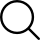Categories

• a
• b
• c
• d
• e
• f
• g
• h
• i
• j
• k
• l
• m
• n
• ñ
• o
• p
• q
• r
• s
• t
• u
• v
• w
• x
• y
• z